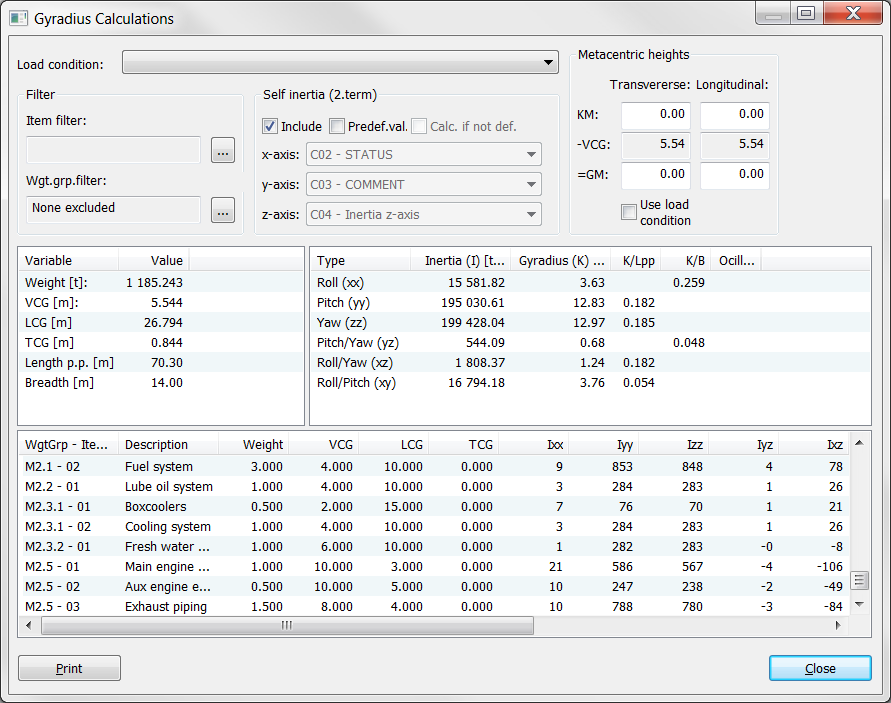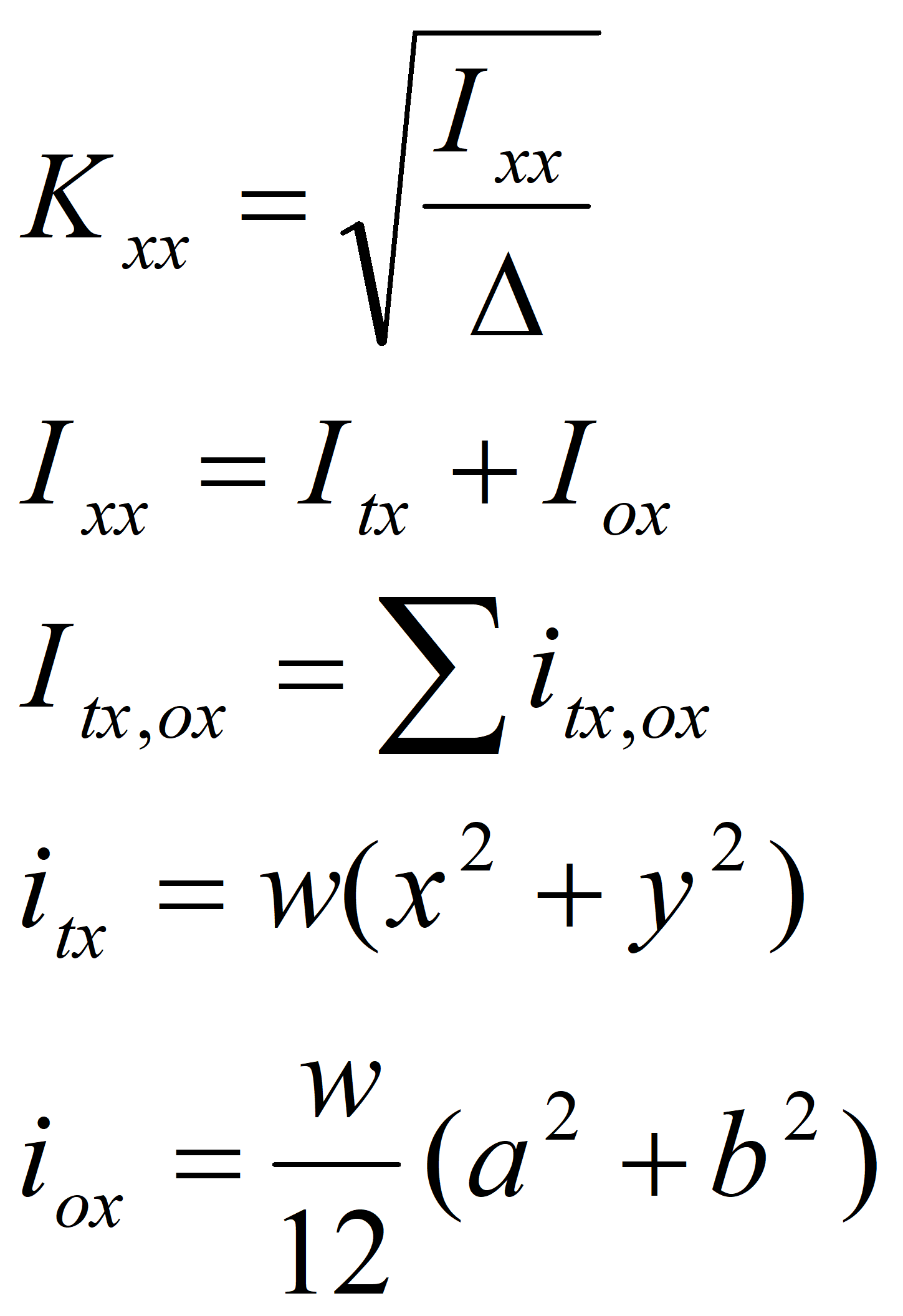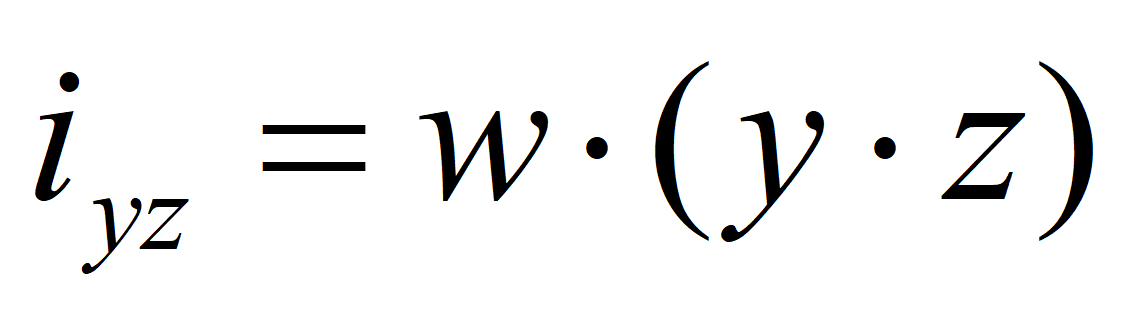The following formulas are used for calculation of Roll gyradius:w = weight of item

Δ = displacement

Itx = sum of transference inertia of elements itx

Iox = sum of item inertia of elements iox

(= 0 when ‘Include 2.term of Steiner theorem’ is not selected)

x,y = location of the item with respect to CoG

a,b = extension of the item

Similar formulas are used for Pitch and Yaw calculations. For Pitch/Yaw calculations, the following formula applies:Similar formulas are used for Roll/Yaw and Roll/Pitch calculations.

If Include 2.term of Steiner theorem is selected, the following rules will apply when calculating moment of inertia:

If a weight item is defined with extension in all 3 dimensions, self-inertia will be calculated for that item and included in the total moment of inertia

If a weight item is defined without extension in all 3 dimensions, self-inertia will be neglected for that item.

When calculating the self-inertia, the shape of the weight item will be approximated to a box

The Gyration window buttons Item filter and Wgt.Grp. filter enables the calculation of gyration for parts of the vessel defined by the filter specifications.  Clicking these buttons will pop up the standard filter window as used in Items window and Weight Distribution window.

The Gyration window will list gyration contribution from each single weight item in the details list of the lower part of the window.  The canned print report from this window also has the option of whether or not to include the item details in the report.

The Gyration window gives the opportunity to include self-inertia defined in custom codes by checking the Predef.Val. checkbox and referring to the custom codes holding self-inertia values by selecting these from the corresponding dropdown lists x, y, and z –axis.